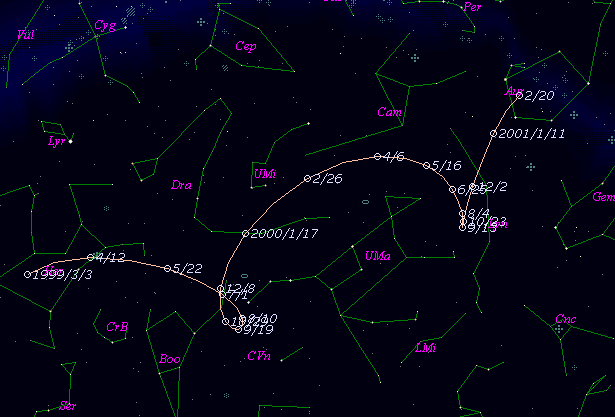# \$B%j%K%"WB@1(B

C/1999 J4 ( LINEAR )

 English version Home page Updated on May 27, 2001###\$B%W%m%U%#!<%k(B

 \$BH/8+F|(B 1999\$BG/(B 5\$B7n(B15\$BF|(B \$BH/8+8wEY(B 18.2-18.8\$BEy(B \$BH/8+ Lincoln Laboratory Near-Earth Asteroid Research project

###\$B50F;MWAG(B

```      Orbital elements by K. Muraoka,
from 39 observations    1999 May 15  to  June 11,
m.e.  =  +/- 0.62 arc seconds.

T  =  1999 Nov. 17.6097  TT
Peri. =   95.1593
Node  =  264.4841   (2000.0)
Incl. =  118.9108
q  =    3.780395  AU
e  =    1.0
```

###\$B@1?^(B###\$B8wEYJQ2=(B

```        m1 = 9.3 + 5 log\$B&\$(B + 10.0 log r
```##### \$B50F;MWAG\$OB<2,7r<#;a\$N7W;;\$K\$h\$k\$b\$N\$G\$9!#(B \$B@1?^\$O(B StellaNavigator Ver.2.0 for Windows (\$B%"%9%H%m%"!<%D(B \$BJTCx(B / \$B%"%9%-!<=PHG6I4)(B) \$B\$G:n@.\$7\$?\$b\$N\$G\$9!#(B \$B8wEY%0%i%U\$O(BComet for Windows\$B\$G:n@.\$7\$?\$b\$N\$G\$9!#(B